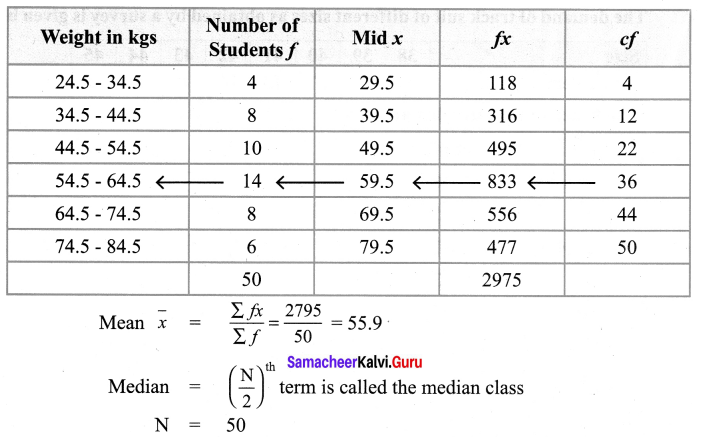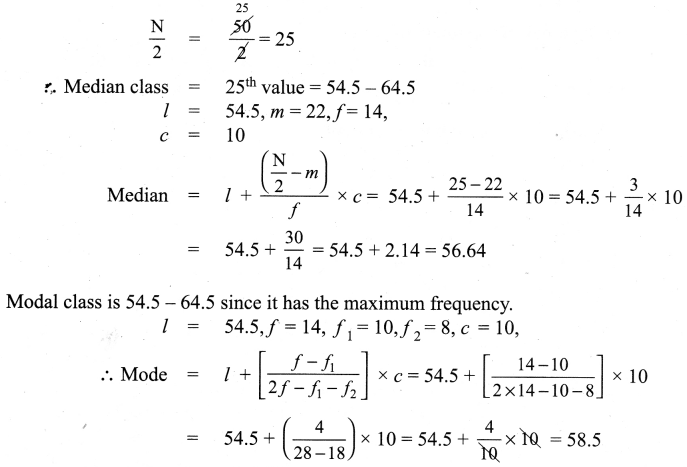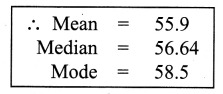# Samacheer Kalvi 9th Maths Solutions Chapter 8 Statistics Ex 8.3

## Tamilnadu Samacheer Kalvi 9th Maths Solutions Chapter 8 Statistics Ex 8.3

Question 1.
The monthly salary of 10 employees in a factory are given below : ₹ 5000, ₹ 7000, ₹ 5000, ₹ 7000, ₹ 8000, ₹ 7000, 17000, ₹ 8000, ₹ 7000, ₹ 5000 Find the mean, median and mode.
Solution:
The monthly salary of 10 employees are ₹ 5000, ₹ 7000, ₹ 5000, ₹ 7000, ₹ 8000, ₹ 7000, ₹ 7000, ₹ 8000, ₹ 7000, ₹ 5000.
Writing in ascending order ₹ 5000, ₹ 5000, ₹ 5000, ₹ 7000, ₹ 7000, ₹ 7000, ₹ 8000, ₹ 8000
Number of values =10 which is an even number.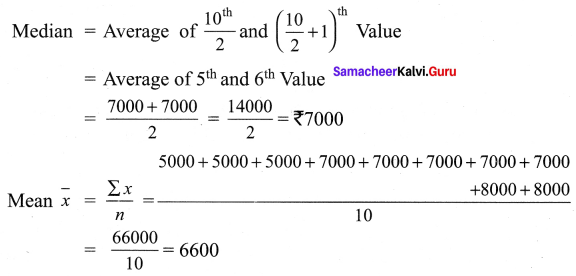In this given data ₹ 7000 occurs maximum number of 5 times
∴ Mode = ₹ 7000/-
∴ Mean = ₹ 6600/-
Mode = ₹ 7000/-Question 2.
Find the mode of the given data : 3.1, 3.2, 3.3, 2.1,1.3, 3.3, 3.1
Solution:
3.1, 3.2, 3.3, 2.1, 1.3, 3.3, 3.1
In this given data 3.1, 3.3 occurs twice
₹ Mode = 3.1 and 3.3 (bimodal)

Question 3.
For the data 11, 15, 17, x + 1, 19, x – 2, 3 if the mean is 14 , find the value of x. Also find the mode of the data.
Solution:
The data given is 11, 15, 17, x + 1, 19, x – 2, 3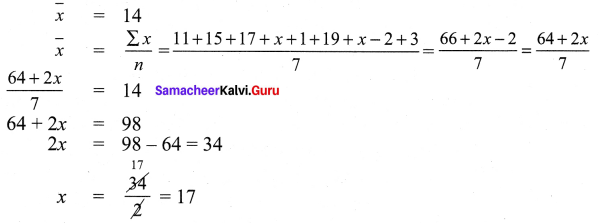The data 11, 15, 17, 17 + 1, 19, 17 – 2, 3 = 11, 15, 17, 18, 19, 15, 3
In this given data 15 occurs twice. Hence the mode is 15.

Question 4.
The demand of track suit of different sizes as obtained by a survey is given below: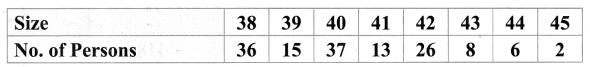Which size is in greater demand ?
Solution: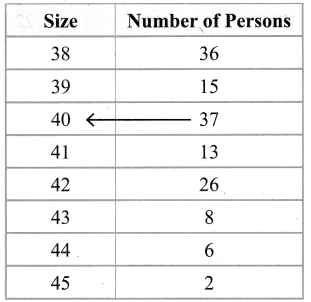∴ Size 40 has maximum frequency 37.
∴ 40 is the mode.Question 5.
Find the mode of the following data: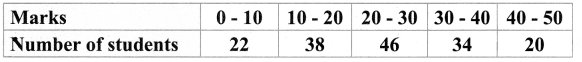Solution: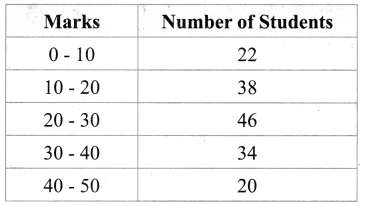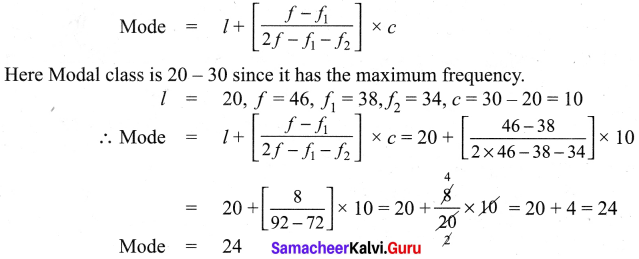Question 6.
Find the mode of the following distribution: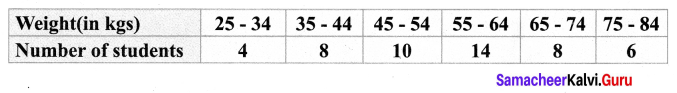Solution: